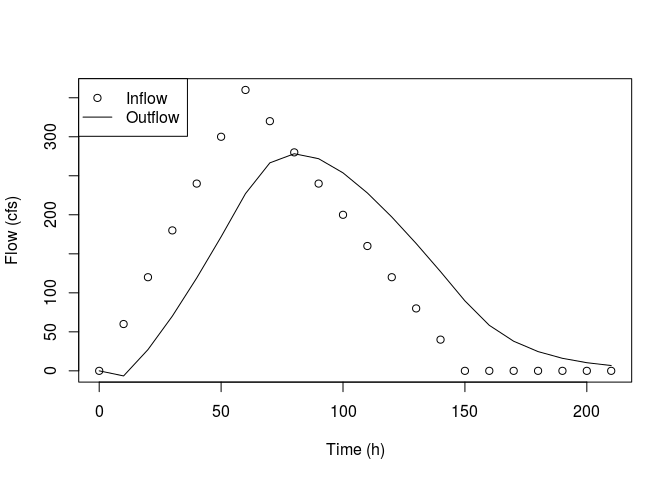# Routing reservoir input hydrographs using the level pool routing method

Level pool routing refers to one of the more straight-forward methods for calculating reservoir outflow given an input hydrograph (time vs inflow) along with information about basin discharge relative to elevation. Here the term reservoir is used in the technical engineering context and does not exclude the use of this method for natural lakes. I believe this method is applicable to any waterbody where you can assume a one-to-one relationship between discharge and elevation. Said another way, we assume that discharge does not have a hysteresis component and does not depend on the direction (rising vs falling limb) of the input hydrograph.

For more a more rigorous mathematical treatment of this method see here, here, and here. The following demonstration will lay out the computational details and describe code to implement the method on three test datasets from the above links.

## Computation

The level pool routing computation procedure involves:

• Determining the volume of the reservoir at a range of depths (if not known ahead-of-time) given its area.

• Developing a relationship between outflow and reservoir-change-in-storage.

• The reference examples use the classical approach of linear interpolation. Here I use non-linear generalized additive modelling to more flexibly represent the shape of this relationship.

Then for each time-step we:

• Calculate the sum of inflows for the current and previous time-steps.

• Calculate change-in-storage-with-time as a function of outflow during the previous time-step.

• Use our fitted relationship to calculate outflows for the current time-step as a function of reservoir change-in-storage.

• Subtract these outflows from the running reservoir storage term.

#' level_pool_routing
#' @param lt data.frame with time and inflow columns
#' @param qh data.frame with elevation and discharge columns.
#'  Storage column optional.
#' @param area numeric reservoir area
#' @param delta_t numeric time step interval in seconds.
#' @param initial_outflow numeric
#' @param initial_storage numeric
#' @param linear.fit logical operator specifying a linear
#'  relationship between outflow and reservoir-change-in-storage
#' @importFrom mgcv gam

level_pool_routing <- function(lt, qh, area, delta_t,
initial_outflow, initial_storage,
linear.fit){

lagpad <- function(x, k) {
c(rep(NA, k), x)[1 : length(x)]
}

lt$ii <- apply(cbind(lagpad(lt$inflow, 1), lt$inflow), 1, sum) if(is.null(qh$storage)){
qh$storage <- area * qh$elevation
}

qh$stq <- ((2 * qh$storage) / (delta_t)) + qh$discharge lt$sjtminq   <- NA
lt$sj1tplusq <- NA lt$outflow   <- NA
lt[1, c("sj1tplusq")] <- c(NA)
lt[1, c("sjtminq")] <- ((2 * initial_storage) / delta_t) -
initial_outflow

lt[1, "outflow"] <- initial_outflow

if(linear.fit == TRUE){
fit <- lm(discharge ~ stq, data = qh)
}else{
fit <- mgcv::gam(discharge ~ s(stq, k = 3), data = qh)

plot(qh$stq, qh$discharge, xlab = "Change-in-storage-with-time",
ylab = "Discharge")
lines(qh$stq, predict(fit)) } for(i in seq_len(nrow(lt))[-1]){ lt[i, "sj1tplusq"] <- lt[i-1, "sjtminq"] + lt[i, "ii"] lt[i, "outflow"] <- predict(fit, data.frame(stq = lt[i, "sj1tplusq"])) lt[i, "sjtminq"] <- lt[i, "sj1tplusq"] - (lt[i, "outflow"] * 2) } lt }  ## Example 1 ### Linear relationship between discharge and change-in-storage The data for this example comes from this level-pool routing walkthrough. We are given an inflow hydrograph in 6 hour increments so we will specify a delta_t timestep of 6 * 3600 seconds. The problem statement specifies an intial storage of 0 and an initial outflow of 20. There is no need to specify reservoir area because we are given storage as a function of discharge. input_hydro <- data.frame( time = seq(0, 162, by = 6), inflow = c(0, 50, 130, 250, 350, 540, 735, 1215, 1800, 1400, 1050, 900, 740, 620, 510, 420, 320, 270, 200, 150, 100, 72, 45, 25, 10, 0, 0, 0)) reservoir_char <- data.frame( elevation = c(130:134, 136:139), discharge = c(20, 34, 57, 96, 162, 463, 781, 1318, 2226), storage = c(1, 1.69, 2.85, 4.8, 8.12, 23.1, 39.1, 65.9, 111)) reservoir_char$storage <- reservoir_char$storage * 1000000  delta_t <- 6 * 3600 res_linear <- level_pool_routing(input_hydro, reservoir_char, area = NA, delta_t = delta_t, initial_outflow = 20, initial_storage = 0, linear.fit = FALSE)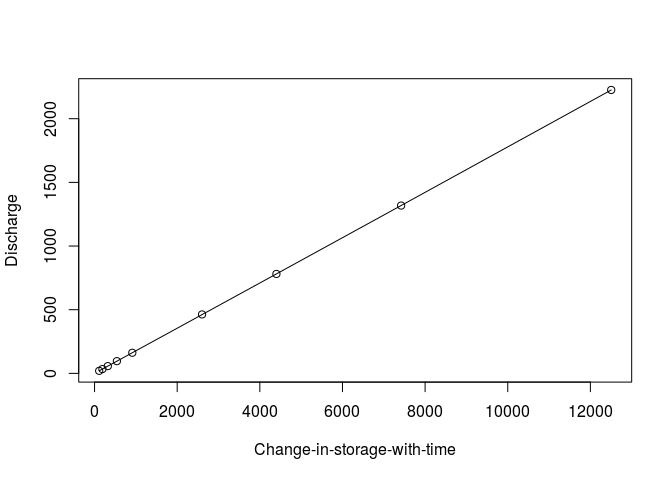plot(res_linear$time, res_linear$inflow, xlab = "Time (h)", ylab = "Flow (m3/s)") lines(res_linear$time, res_linear$outflow) legend("topleft", legend = c("Inflow", "Outflow"), lty = c(NA, 1), pch = c(21, NA))## Example 2 ### Curvilinear relationship between discharge and change-in-storage The data for this example comes from this level-pool routing walkthrough pdf. I scraped the data tables from the pdf using the pdftools package. We are given storage as a function of discharge so we have no need for information on the area of the reservoir. Also, we are given an inflow hydrograph in 2 hour increments so we will specify a delta_t timestep of 2 * 3600 seconds. Unlike the previous example, our reservoir has a non-zero initial storage. library(pdftools) txt <- strsplit(pdf_text( "https://www.caee.utexas.edu/prof/maidment/CE374KSpr12/Docs/Hmwk5Soln.pdf"), "\n")[] parse_table <- function(tbl, tbl_names){ tbl <- lapply(tbl, function(x) read.table(text = x, stringsAsFactors = FALSE)) tbl <- lapply(tbl, function(x) gsub(",", "", x)) inds <- lapply(tbl, function(x) ifelse(max(grep(")", x)) == -Inf, 1, max(grep(")", x)))) inds <- lapply(seq_along(tbl), function(x) c(1, (inds[[x]] + 1):length(tbl[[x]]))) tbl <- lapply(seq_along(tbl), function(x) as.numeric(tbl[[x]][inds[[x]]])) tbl <- data.frame(t(do.call("rbind", tbl))) tbl <- tbl[2:nrow(tbl),] names(tbl) <- tbl_names tbl } tbl1 <- suppressWarnings(rbind( parse_table(txt[6:7], tbl_names = c("time", "inflow")), data.frame(time = seq(20, 36, by = 2), inflow = 0))) tbl2 <- suppressWarnings( parse_table(txt[9:10], tbl_names = c("storage", "discharge"))) tbl2$storage <- tbl2$storage * 1000000  res_curv <- level_pool_routing(lt = tbl1, qh = tbl2, area = NA, delta_t = 7200, initial_outflow = 57, initial_storage = 75000000, linear.fit = FALSE)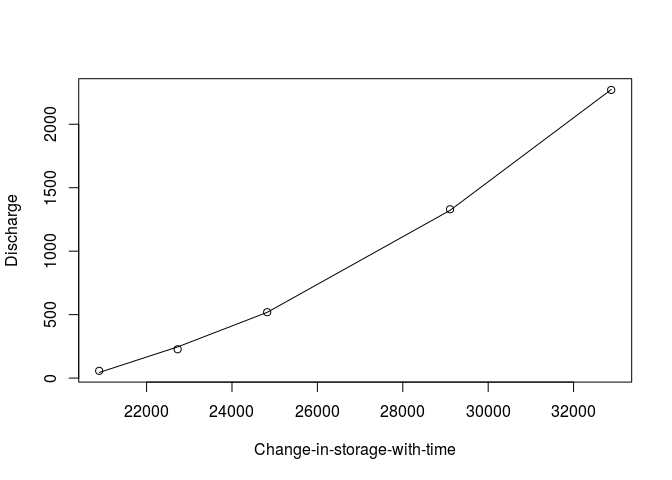plot(res_curv$time, res_curv$inflow, xlab = "Time (h)", ylab = "Flow (m3/s)") lines(res_curv$time, res_curv$outflow) legend("topleft", legend = c("Inflow", "Outflow"), lty = c(NA, 1), pch = c(21, NA))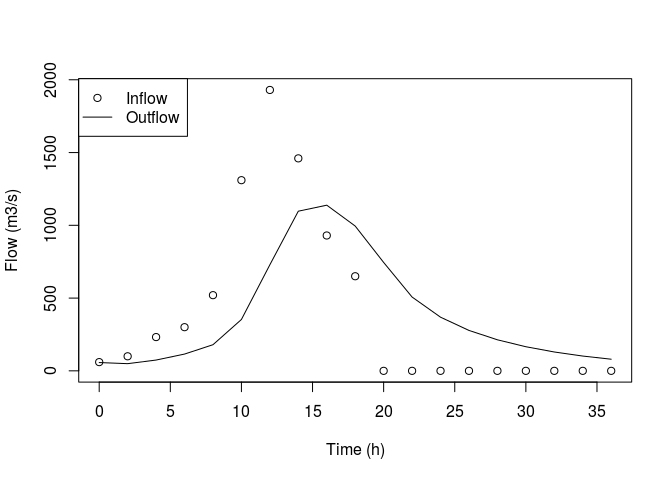## Example 3 ### Oscillating relationship between discharge and change-in-storage The data for this example comes from this level-pool routing walkthrough ppt. Here we are given discharge as a function of reservoir elevation but we are not given the corresponding storage. As a result, we must specify a reservoir area. We are given an inflow hydrograph in 10 minute increments so we will specify a delta_t timestep of 10 * 60 seconds. In this case, our reservoir a zero initial storage and initial outflow. lt <- data.frame(time = seq(0, 210, by = 10), inflow = c(seq(0, 360, by = 60), seq(320, 0, by = -40), rep(0, 6))) qh <- data.frame(elevation = seq(0, 10, by = 0.5), discharge = c(0, 3, 8, 17, 30, 43, 60, 78, 97, 117, 137, 156, 173, 190, 205, 218, 231, 242, 253, 264, 275))  res_osc <- level_pool_routing(lt, qh, area = 43560, delta_t = 600, initial_outflow = 0, initial_storage = 0, linear.fit = FALSE)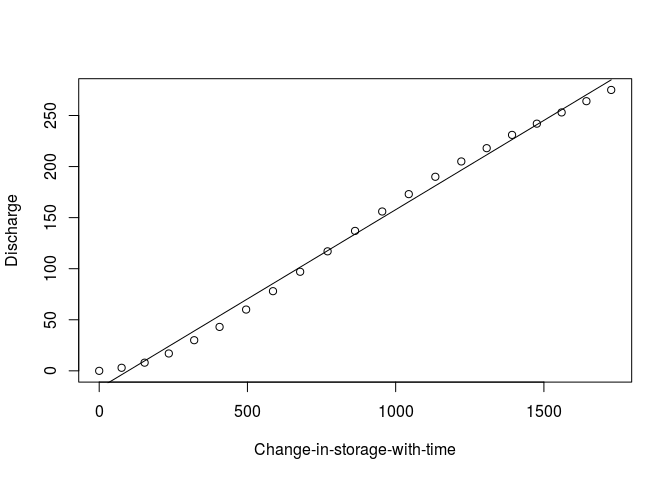plot(res_osc$time, res_osc$inflow, xlab = "Time (h)", ylab = "Flow (cfs)") lines(res_osc$time, res_osc\$outflow)
legend("topleft", legend = c("Inflow", "Outflow"), lty = c(NA, 1),
pch = c(21, NA))